# SSC CGL EXAMS 2019 | Reasoning Ability Practice Questions (Day-22)

Dear Aspirants, Here we have given the Important SSC Exam 2019 Practice Test Papers. Candidates those who are preparing for SSC 2019 can practice these questions to get more confidence to Crack SSC 2019 Examination.

[WpProQuiz 4920]

1) Which one of the following diagrams represents correct relationship among sparrow, birds, and Kite?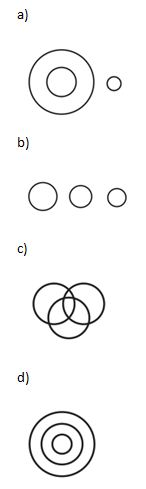2) How many meaningful English words can be made with the letter’s ‘X Y E NL O’, using each letter only once in each word?

a) Two

b) Three

c) One

d) More than Three

3) If 1 bird from group 1 is come to group number 2, then the group – 2 has twice the number of birds that in group 1 has. If 1 bird from group 2 is come to group – 1, the group – 2 and group – 1 have to same numbers of birds. How many birds were there in group I and group II and what is their G.M?

a) 5, 7, √35

b) 7, 5, √35

c) √7, 5, 35

d) None of these

4) Ramesh is taller than Arun who is shorter than Sunil. Guru is taller than Santra but shorten than Arun. Sunil is shorter than Ramesh. Who is the tallest?

a) Ramesh

b) Arun

c) Guru

d) Sunil

5)  What should come in place of?

5 6 K 3 1 D 3 3 F? 2 G

a) 0

b) 1

c) 5

d) 3

6) Find the missing number?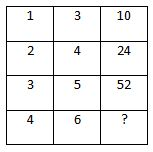a) 100

b) 28

c) 27

d) 101

7) In a certain code language –

A * B denotes A is father of B

A – B denotes A is mother of B

A÷B denotes A is brother of B

A ×B denotes A is sister of B

In, P ÷Q×R * S, How P is related to S?

8)  Find the next term of the given series—

51, 52, 48, 57, 42, ?

1) 56

2) 66

3) 36

4) 63

9)  How many such letters are there is the word ‘PSYCHOPHANT’ each of which is as far away from the beginning of the word as when they are arranged in alphabetical order?

a) None

b) One

c) Three

d) Two

10) Book is written as — ‘13283230’ then in the same code language ‘TABLE’ is written as–

a) 3114193128

b) 314139128

c) 3224293168

d) None of these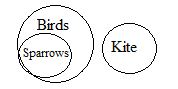Words can be formed using the letters “XYENLO” – No, on, oxen and ox.

X – 1 = y + 1

Y + 1 = 2*2

Y – 2x = – 3

Y = 2x – 3

We put the value of ‘y’ in eqs. (i) we, get,

2x – 3 –1= x + 1

x = 1 + 3 + 1 = 5

y = 5×2 – 3 = 7

Ramesh, Sunil > Arun

Arun > Guru > Santra

Ramesh > Sunil

Ramesh > Sunil > Arun > Guru> Santra.

So, Ramesh is the tallest.

5 + 6 = 11 = K; 3 + 1 = 0 4 = D;

3 + 3 = 06 = F; 5 +2 = 07 = G

13 + 32 = 10; 23 + 42 = 24

= 33 + 52 = 52

So, 43 + 62 = 100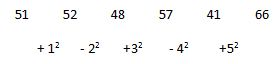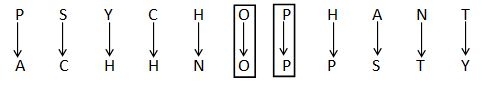Here we odd different prime number in each letter starting from the.

B => 2 + 11 = 13

O => 15 + 13= 28

O => 15 + 17= 32

K => 11 + 19= 30

BOOK = 13283230

Similarly,

T => 20 + 11 = 31

A => 1 + 13 = 14

B => 2 + 17 = 19

L => 12 + 19 = 31

E => 5 + 23 = 28

TABLE = 3114193128

*********************************